# A raindrop

A raindrop falls at a rate of 9.3 meters per second (m/s). Use the following facts to convert this speed to kilometers per hour (km/h).

1 km = 1000 m

1 min = 60 sec

1 hour = 60 min

v =  33.48 km/h

### Step-by-step explanation: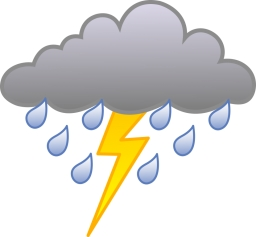Did you find an error or inaccuracy? Feel free to write us. Thank you!Tips to related online calculators
Do you want to convert length units?
Do you want to convert velocity (speed) units?
Do you want to convert time units like minutes to seconds?

## Related math problems and questions:

• Mail trainThe speed of the mail train is 1370 meters per minute. Express it in miles per hour, correct to three significant figures. Use the following facts to convert this units: 1 meter =39.37 inches, 1 mile = 1609 m, 1 hour = 60 minutes
• Motion problemFrom Levíc to Košíc go car at speed 81 km/h. From Košíc to Levíc go another car at speed 69 km/h. How many minutes before the meeting will be cars 27 km away?
• Cheetah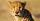Cheetah elapses 150 m for 5 seconds, the eagle reaches speeds up to 230 km/h, dolphin swims 1.2 km per minute. Which animal is the fastest and slowest? The results write as meters per second (m/s).
• The cyclistThe cyclist drove from A to B, 60 km away. The first half drove at 30km/h, the second at 20km/h. How long did it take for him?
• Cyclist 9A cyclist travels at a speed of 4.25 km per hour. At that rate, how far can he travel in 3.75 hours?
• Aircraft nose downHow long will fall airliner from a height of 10000 m at speed 1,000 km/h?
• Average speed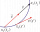The car drove on one section of the highway for half an hour at a speed of 80 km/h. Then he reduced his speed to 60km/h. It went three-quarters of an hour at a speed of 60 km/h. What was the average speed of the car?
• Cyclist 12What is the average speed of a cycle traveling at 20 km in 60 minutes in km/h?
• Speed of sound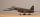The average speed of sound is 330 meters per second. Estimate how long it will take to hear the church bell 1 km away. Calculate the distance from what would hear sound after 10 seconds.
• MotionIf you go at speed 3.7 km/h, you come to the station 42 minutes after leaving the train. If you go by bike to the station at speed 27 km/h, you come to the station 56 minutes before its departure. How far is the train station?
• Train from BrnoFirst train started from Brno at 8 am at 60 km per hour. Second train started from Opava at 7 o'clock at the speed of 40 kilometers per hour. In how many hours and how far from cities trains meet when the distance of cities is 200 kilometers.
• Two trains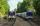Two trains go at each other. First at speed 40 km/h and the second at speed 60 km/h. At what distance will be 30 minutes before they will meet?
• Two cars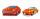From the town A to town B started two cars. The first at 7:00 at average speed 60 km per hour, the second at 10:00 at average speed 100 km per hour. The first car will not stay in B, and on the way back meet the second car at half way from A to B. At what
• GrandmotherMom walked out to visit her grandmother in a neighboring village 5km away and moved at a speed of 4km/h. An hour later, father drove down the same road at an average speed of 64km/h. 1) How long will take to catch mom die? 2) What is the approximate dista
• Dog II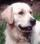The journey from a rangers' home to the town is 6 km. Mr. ranger started from his home and went 3 km per hour. His women came from the town and also went 3 km per hour. With ranger started dog and runs in 12 km in 1 hour. The dog went to the ranger's woma
• Pedestrian and cyclist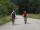Pedestrian out with a speed of 4 km/hour from city center and after 1hour and 10 minutes came after him cyclist at speed of 18 km/h. At how many minutes he catches up with pedestrian?
• Motion 2At 10 o'clock started from place A car speed at 90 km/h. Twenty minutes after starting another car from place B at a speed 60 km/h . Distance places A and B is 120 kilometers. What time and where do they meet?